# Replacing Cell References in Multiple Cells (Formulas)

This post will guide you how to replace a cell reference in multiple cells in excel. How do I replace Cell or Sheet reference in copied formulas in your worksheet in excel. How to quickly find and replace a cell reference in excel formula. How to replace references in formula with another Cell reference or value in excel. And this post will explain that how to find and replace part of formula in all cells in excel.

## Replacing Cell References

Assuming that you have applied the following formula in cell C1, and then copied this formula over other cells.

` =DATE(\$B\$1,1,1)+CHOOSE(WEEKDAY(DATE(B1,1,1),2),0,6,5,4,3,2,1)`

If you want to change part of formula for all copied formula, for example, changing one cell reference \$B\$1 to \$A\$1, you can use the Find and Replace feature to achieve it. Just do the following steps:

#1 select the range of cells that you have applied this formula.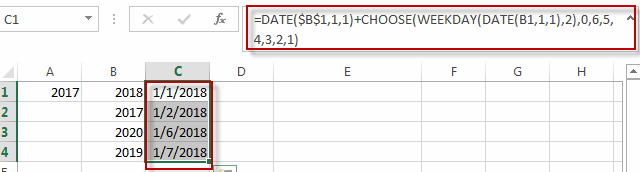#2 go to HOME tab, click Find & Select command under Editing group, then select Replace… from the drop-down menu list, the Find and Replace dialog will appear.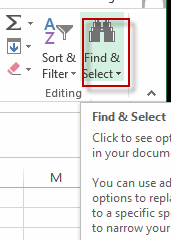#3 type \$B\$1 into the text box of Find what, and type \$A\$1 into the text box of Replace with box. Then click Options<< button.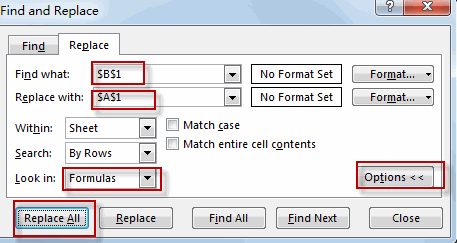#4 select Formulas from the Look in list box, then click Replace All button.

#5 click OK button.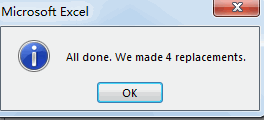#6 let’s see the result.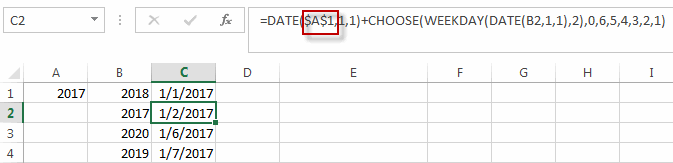You will see all cell references have been replaced with another cell reference in all copied formulas.

### Related Functions

• Excel DATE function
The Excel DATE function returns the serial number for a date.The syntax of the DATE function is as below:= DATE (year, month, day)…
• Excel Choose Function
The Excel CHOOSE function returns a value from a list of values. The CHOOSE function is a build-in function in Microsoft Excel and it is categorized as a Lookup and Reference Function.The syntax of the CHOOSE function is as below:=CHOOSE (index_num, value1,[value2],…)…